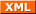#tianjara.net |Andrew Harvey's Blog

### Entries from August 2008.

30th August 2008

Many of the courses I have studied so far (such as HSC Mathematics and 1st year MATH1131 and MATH1231 [UNSW]) require some form of mathematical proofs to be used. However in these courses they don't really teach us about proof's, rather they tend to say here is the generic proof to use, memorise it and write it out in the exam. They don't always do this, but most of the time they have asked us to do a proof in an exam there is a similar example provided in the notes somewhere.

I have always found doing proof questions very hard and I think the main reason is that they never actually taught us them! Lucky for me I'm doing MATH1081 which has a section all about proof and I must say, I've learnt a lot of very important and interesting things from this course.

A lot of the time when I saw proofs I would think to myself, that is not a proof! Mainly either because it was so obvious that you can just see its true, or because the proof says its true because of such and such reason, with no explanation as to why such and such reason was also true.

However now that I've been taught proofs, things are starting to make sense. Proofs are actually quite simply, they are there just to convince the reader that some result is true based on some agreed upon facts. Proofs just build on things to provide new results based on those results already known. It is quite acceptable to not prove certain things in a proof because they themselves have proofs and if you were to include these proofs every time you find a new result you would have to publish a whole new book with a couple extra pages stuck on the back.

This whole idea of having proof's based on axioms/postulates is very interesting. In Euclid's Elements, Euclid first writes down a series of definitions so that he has something to base his postulates and theorems on. Without first accepting these basic facts nothing can be proved. Some people say that these axioms are obviously true, I tend to disagree. Rather than viewing them are true or false I think it would be more appropriate to not label them as either. They are merely axioms on which you base deductions. I also think you should not just consider things that are based on these axioms. It would of course be very interesting to investigate the mathematics if you have some very weird axioms that most would say are obviously false. Again I would say its not a matter of this fact being true and this being false, rather here is a statement and here is what you can deduce from that statement.

Back to the idea of what is considered a proof, I think my lecturer has explained it well. A proof is merely a argument, something which aims to convince the reader that the statement which you are proving is in fact true based on those agreed upon facts. You simply show how to obtain the result from simpler things which one also accepts to be true.

Another concept which came up was the use of diagrams in proofs. When we studied sets and we had a question which said prove A is a subset of B, I always thought that the easiest way to show this was to draw a Venn diagram and show that A is always inside B. Then again you could always argue that you are just drawing a diagram of the result you are trying to prove!

From an education perspective, I think the very lack of teaching mathematical proof all the way up until math1081 and yet expecting us to understand proofs is completely stupid. If we ever want to have a deeper understanding of the things we learn and know why they work, then we should be taught the fundamentals first!

Now, I'm sure I've said some things which are probably not entirely correct. I accept that, I'm not an expert in maths, so don't expect me to.

Update (18th Oct 2008): I just read this in a maths text book,

If $x+3=5,$ then $(x+3) + (-3) = 5 + (-3);$ hence $x + (3 + (-3)) = 5 - 3 = 2;$ hence $x + 0 = 2$; hence $x = 2.$

They go on to say,

"Naturally, such elaborate solutions are of interest only until you become convinced that they can always be supplied. In practice, it is usually just a waste of time to solve and equation by indicating so explicitly the reliance on (the laws of elementary algebra)."

I think this is the best example of what my troubles were in understanding the whole concept of proof. I guess the largest challenge that I face now is in examinations how do I know what level of maths I can assume the examiner agrees upon, and how much I actually have to prove.

References:  Spivak, M. (1973). Calculus. Addison-Wesley. pp 4-5.

Tags: education, math1081, mathematics.